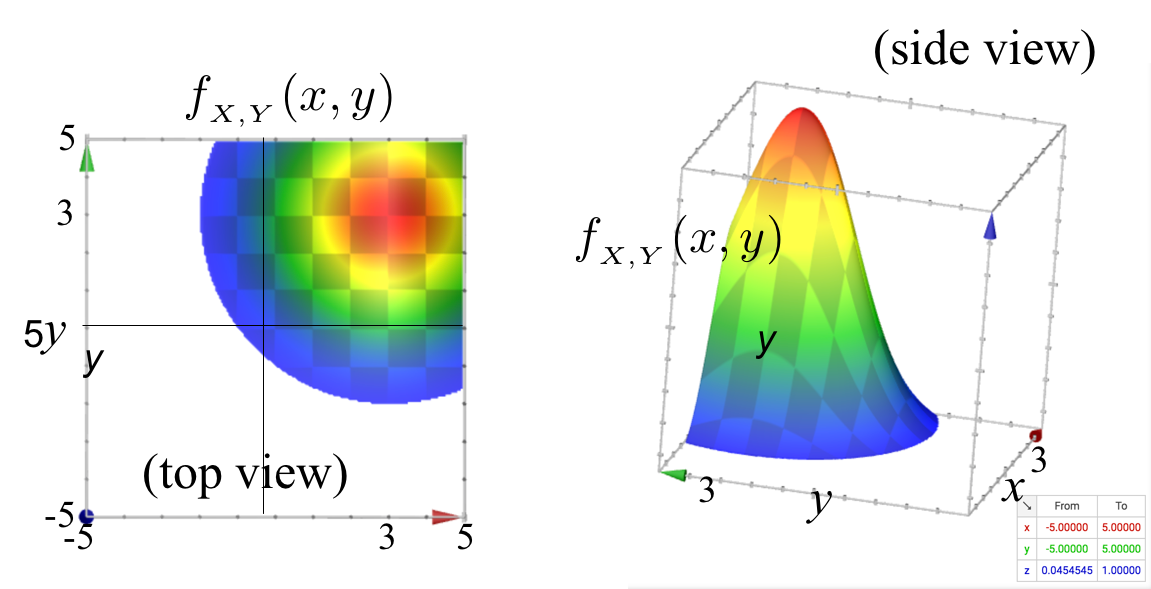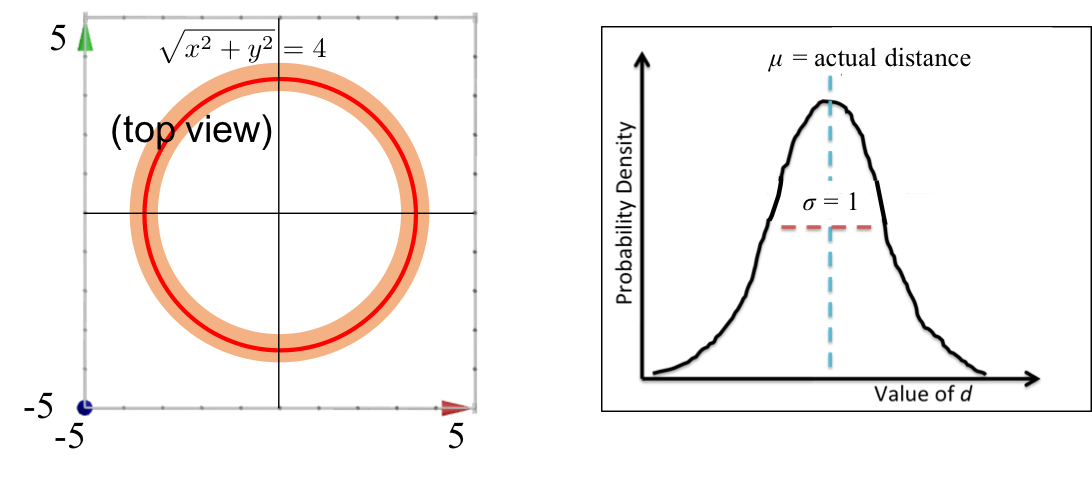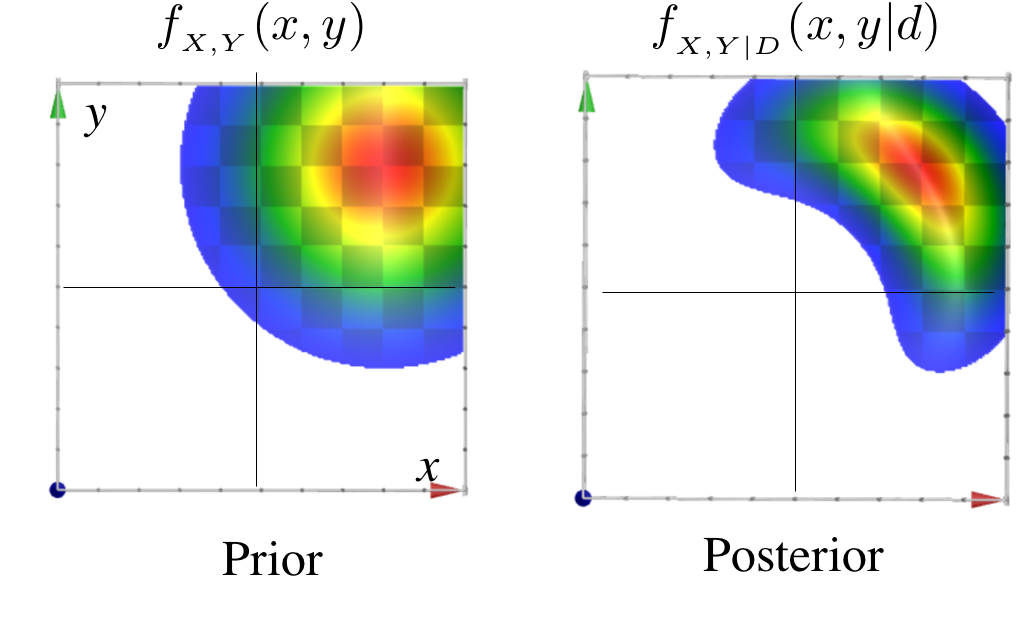# Tracking in 2D

Warning: After learning about joint distributions, and about inference, you have all the technical abilities necessary to follow this example. However this is very very difficult. Primarily because you need to understand three complex things at the same time: (1) how to represent a continuous joint distribution, (2) inference in probabilistic models and (3) a rather complex probability of observation calculation.

In this example we are going to explore the problem of tracking an object in 2D space. The object exists at some $(x, y)$ location, however we are not sure exactly where! Thus we are going to use random variables $X$ and $Y$ to represent location. \begin{align*} f(X = x, Y = y) &= f(X = x) \cdot f(Y = y) && \text{In the prior X and Y are independent} \\ &= \frac{1}{\sqrt{2 \cdot 4 \cdot \pi}}\cdot e ^{-\frac{(x-3)^2}{2 \cdot 4}} \cdot \frac{1}{\sqrt{2 \cdot 4 \cdot \pi}}\cdot e ^{-\frac{(y-3)^2}{2 \cdot 4}} && \text{Using the PDF equation for normals} \\ &= K_1 \cdot e ^{-\frac{(x-3)^2 + (y-3)^2}{8}} && \text{All constants are put into } K_1 \end{align*} This combinations of normals is called a bivariate distribution. Here is a visualization of the PDF of our prior.The interesting part about tracking an object is the process of updating your belief about it's location based on an observation. Let's say that we get an instrument reading from a sonar that is sitting on the origin. The instrument reports that the object is 4 units away. Our instrument is not perfect: if the true distance was $t$ units away, than the instrument will give a reading which is normally distributed with mean $t$ and variance 1. Let's visualize the observation:Based on this information about the noisiness of our prior, we can compute the conditional probability of seeing a particular distance reading $D$, given the true location of the object $X$, $Y$. If we knew the object was at location $(x, y)$, we could calculate the true distance to the origin $\sqrt{x^2 + y^2}$ which would give us the mean for the instrument Gaussian: \begin{align*} f(D = d | X = x, Y = y) &= \frac{1}{\sqrt{2 \cdot 1 \cdot \pi}}\cdot e ^{-\frac{\big(d-\sqrt{x^2 + y^2}\big)^2}{2 \cdot 1}} && \text{Normal PDF where } \mu = \sqrt{x^2 + y^2} \\ &= K_2\cdot e ^{-\frac{\big(d-\sqrt{x^2 + y^2}\big)^2}{2 \cdot 1}} && \text{All constants are put into } K_2 \end{align*} How about we try this out on actual numbers. How much more likely is an instrument reading of 1 compared to 2, given that the location of the object is at (1, 1)? \begin{align*} \frac{f(D = 1 | X = 1, Y = 1)}{f(D = 2 | X = 1, Y = 1) } &= \frac {K_2\cdot e ^{-\frac{\big(1-\sqrt{1^2 + 1^2}\big)^2}{2 \cdot 1}}} {K_2\cdot e ^{-\frac{\big(2-\sqrt{1^2 + 1^2}\big)^2}{2 \cdot 1}}} && \text{Substituting into the conditional PDF of D}\\ &= \frac {e ^0} {e^{-1/2}} \approx 1.65 && \text{Notice how the $K_2$ cancel out} \end{align*} At this point we have a prior belief and we have an observation. We would like to compute an updated belief, given that observation. This is a classic Bayes' formula scenario. We are using joint continuous variables, but that doesn't change the math much, it just means we will be dealing with densities instead of probabilities: \begin{align*} f&(X =x, Y =y | D =4) \\ &= \frac{f(D = 4| X = x, Y =y) \cdot f(X = x, Y = y)}{f(D =4)} && \text{Bayes using densities}\\ &= \frac{K_1 \cdot e^{-\frac{[4 - \sqrt{x^2 + y^2})^2]}{2}} \cdot K_2 \cdot e^{-\frac{[(x - 3)^2 + (y - 3)^2]}{8}}}{f(D = 4)} && \text{Substitute}\\ &= \frac{K_1 \cdot K_2}{ { f(D =4)}} \cdot e ^{-\big[\frac{[4 - \sqrt{x^2 + y^2})^2]}{2} + \frac{[(x - 3)^2 + (y - 3)^2]}{8} \big]} && \text{$f(D=4)$ is a constant w.r.t. $(x,y)$}\\ &= K_3 \cdot e ^{-\big[\frac{(4 - \sqrt{x^2 + y^2})^2}{2} + \frac{[(x - 3)^2 + (y - 3)^2]}{8} \big]} && \text{$K_3$ is a new constant} \end{align*} Wow! That looks like a pretty interesting function! You have successfully computed the updated belief. Let's see what it looks like. Here is a figure with our prior on the left and the posterior on the right:How beautiful is that! Its like a 2D normal distribution merged with a circle. But wait, what about that constant! We do not know the value of $K_3$ and that is not a problem for two reasons: the first reason is that if we ever want to calculate a relative probability of two locations, $K_3$ will cancel out. The second reason is that if we really wanted to know what $K_3$ was, we could solve for it. This math is used every day in millions of applications. If there are multiple observations the equations can get truly complex (even worse than this one). To represent these complex functions often use an algorithm called particle filtering.